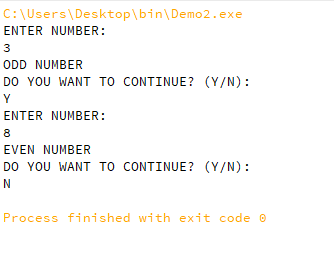# COBOL – Include Statement

• Difficulty Level : Medium
• Last Updated : 24 Mar, 2022

The INCLUDE statement refers to the file or the directory mentioned after it and inserts the command present inside that member in the pre-compilation state. Basically, it is used to insert a code block onto a source program.

Syntax:

`INCLUDE member-name`

Where,

• The member-name refers to the name of the file that has the source code.

Following are the advantages of using the INCLUDE statement:

• Increase the readability of the code and reduce the length of the code.
• Suppose the same code is used repeatedly inside an application instead of writing the same part of code repeatedly we can use Include which will insert that same code inside a program.
• Reduce maintenance cost, time, and efforts when the changes are done only once inside the modules which are included in many programs.

Let’s take an example of the INCLUDE statement in Cobol.

Example:

## Cobol

 `IDENTIFICATION` `DIVISION``. ` `PROGRAM``-``ID``. DEMO2. ` `DATA` `DIVISION``. ` `FILE` `SECTION``. ` `WORKING-STORAGE` `SECTION``. ` `    ``01` `NUM  ``PIC` `9``(``5``) ``VALUE` `ZERO``. ` `    ``01` `REM  ``PIC` `9``(``5``) ``VALUE` `ZERO``. ` `    ``01` `DIV  ``PIC` `9``(``5``) ``VALUE` `ZERO``. ` `    ``01` `FLAG ``PIC` `A(``1``) ``VALUE` `'Y'``. ` `PROCEDURE` `DIVISION``. ` `MAIN-``PROCEDURE``. `   `    ``A-PARA. ` `    ``INCLUDE ODDEVE. ` `    ``EXIT``. `   `    ``PERFORM` `A-PARA ``UNTIL` `FLAG ``EQUAL` `'N'`   `    ``STOP` `RUN``.`

Explanation:

The program DEMO2 is using a member ODDEVE with the help of Include syntax in COBOL, when the program executes instead of line INCLUDE ODDEVE  the actual code present inside the member ODDEVE(shown below) is inserted which is logically written to check whether the number is odd or even.

## Cobol

 `DISPLAY` `'ENTER NUMBER:'` `ACCEPT` `NUM `   `DIVIDE` `NUM ``BY` `2` `GIVING` `DIV ``REMAINDER` `REM. `   `IF` `REM = ``0` `    ``DISPLAY` `'EVEN NUMBER'` `    ``DISPLAY` `'DO YOU WANT TO CONTINUE? (Y/N):'` `    ``ACCEPT` `FLAG `   `ELSE` `    ``DISPLAY` `'ODD NUMBER'` `    ``DISPLAY` `'DO YOU WANT TO CONTINUE? (Y/N):'` `    ``ACCEPT` `FLAG ` `END``-``IF``.`

Output:My Personal Notes arrow_drop_up
Recommended Articles
Page :